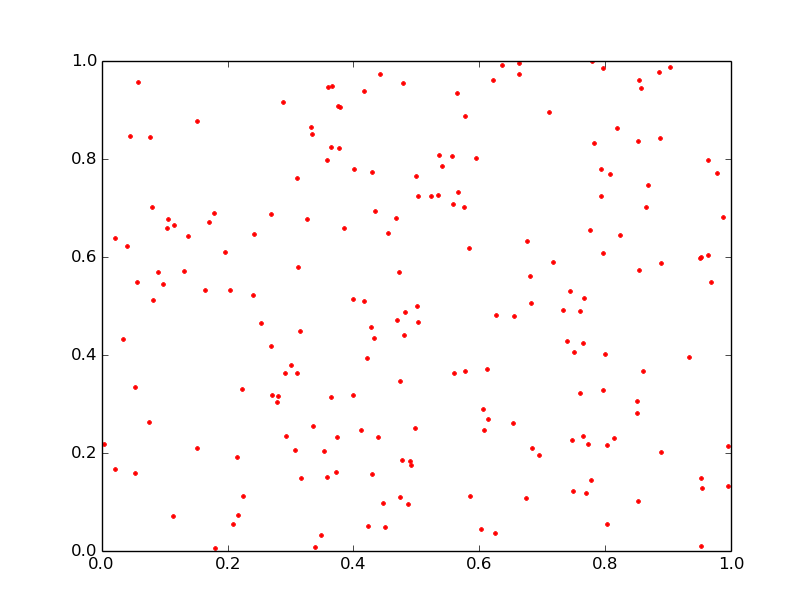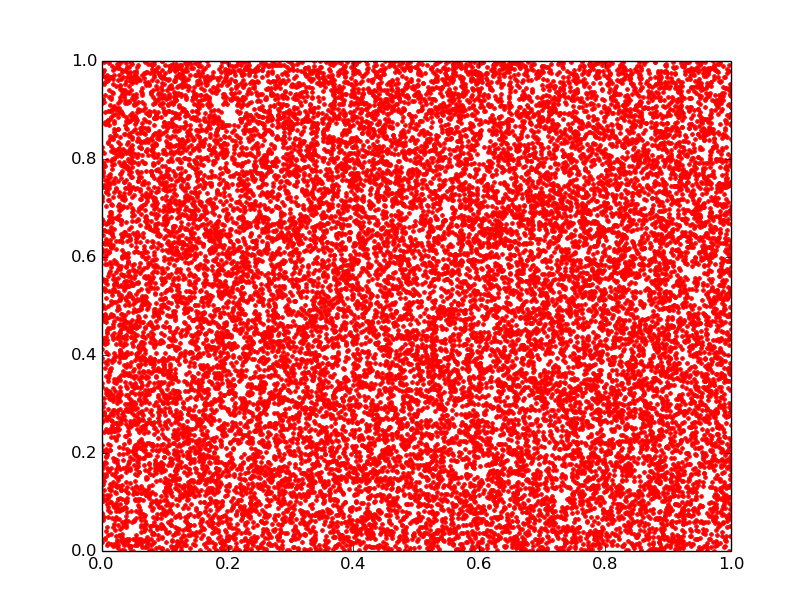# 一个观察随机数是否足够随机的方法

2016/04/11 21:17

import random
import matplotlib.pyplot as plt

points_num = 200
X = []
Y = []
for _ in range(0,points_num):
X.append(random.random())
Y.append(random.random())

plt.plot(X, Y, 'r.')
plt.show()1
3 收藏

### 作者的其它热门文章0 评论
3 收藏
1